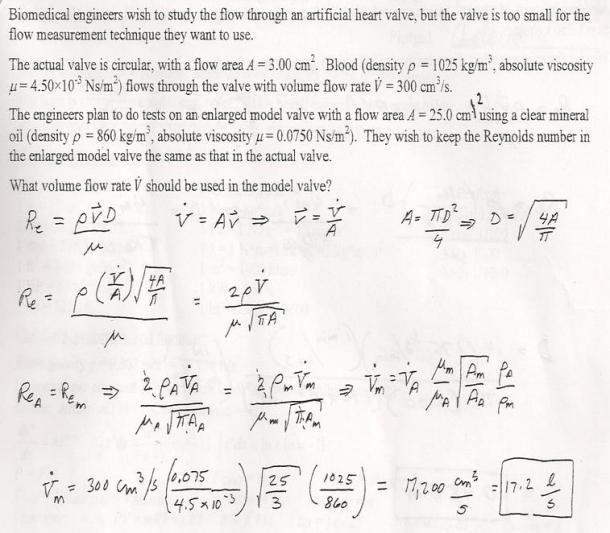fluid mechanics math problems engineering equations fluid mechanics basic mechanics school homework engineering math fluids formulas fluid mechanics problems fluid mechanics problem solutions to fluids problems full solution fluid mechanics engineering fluids problem solution fluid mechanics math problems engineering equations fluid mechanics basic mechanics school homework engineering math fluid mechanics formulas fluid problems fluid mechanics problem solutions to fluid mechanics problems full solution fluid mechanics engineering fluid mechanics problem solution
fluid mechanics math problems engineering equations fluid mechanics basic mechanics school homework engineering math fluids formulas fluid mechanics problems fluid mechanics problem solutions to fluids problems full solution fluid mechanics engineering fluids problem solution fluid mechanics math problems engineering equations fluid mechanics basic mechanics school homework engineering math fluid mechanics formulas fluid problems fluid mechanics problem solutions to fluid mechanics problems full solution fluid mechanics engineering fluid mechanics problem solution
Highalphabet Home Page fluid mechanics solutions fluids math solved Fluid Mechanics Page 1
Biomedical engineers wish to study the flow through an artificial heart valve, but the valve is too small for the flow measurement technique they want to use. The actual valve is circular, with a flow area A=3.00 cm^2. blood flows through the valve with volume flow rate Vdot=300 cm^3/s. The eingineers plan to do tests on an enlarged model valve with a flow area A=25 cm^2 using a clear mineral oil. they wish to keep the Reynolds number in the enlarged model valve the same as that in the actual valve. What volume flow rate should be used in the model valve?Biomedical engineers wish to study the flow through an artificial heart valve, but the valve is too small for the flow measurement technique they want to use. The actual valve is circular, with a flow area A=3.00 cm^2. blood flows through the valve with volume flow rate Vdot=300 cm^3/s. The eingineers plan to do tests on an enlarged model valve with a flow area A=25 cm^2 using a clear mineral oil. they wish to keep the Reynolds number in the enlarged model valve the same as that in the actual valve. What volume flow rate should be used in the model valve?